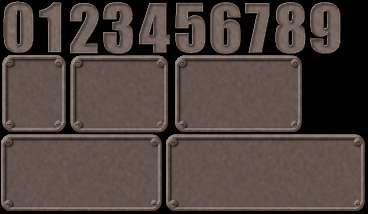# Mixed Number Calculator

Instructions: Use this calculator to compute mixed fractions. Please provide your mixed fraction in the box below.The mixed fraction you want to convert (Ex: 2 3/2)

## How to use this mixed number calculator

This mixed fraction calculator helps you compute any algebra expression involving mixed numbers and fractions that you provide. For example, you can provide a mixed number like '2 3/4' and the calculator will convert it to a regular fraction and it will reduce it.

Once you have provided a mixed number/fraction expression, you need to click on "Calculate", and all the steps will be shown for you.## What is a mixed fraction

A mixed fraction is simply an integer that goes along with a fraction. The format is: the integer goes first, then a space and then a fraction. For example, the following is a mixed fraction:

$2\,\,\frac{2}{3}$

In this case, the integer is '2', and the fraction is '2/3'. Having these two entities together in this case means that we are adding them. This is, when we write the mixed fraction, we mean the following:

$2\,\,\frac{2}{3} = 2 + \frac{2}{3}$

## How do compute mixed numbers

The basic idea is to simple reduce the mixed number to a sum of fractions. This is, you need to separate the integer part and the fraction part of a mixed number and the operate them as regular fractions.

## What are the steps for the calculation of mixed numbers

• Step 1: Identify clearly what is the mixed number we want to analyze
• Step 2: Extract the integer part and the fraction part of the mixed number
• Step 3: Convert the integer part to fraction and then simply operate them as fractions

## Why would deal with mixed fractions?

Using mixed fractions (also known as mixed numbers) is sort of a legacy notation. It actually does not have a notable importance nor does it play any important role. But it is good to know how to operate them, since the pop up in formulas from time to time.### Example: Calculating mixed number

Write as a fraction: $$1\,\,\frac{1}{3}$$.

Solution:

We need to simplify the following given mixed fraction: $$\displaystyle 1 \,\, \frac{ 1}{ 3}$$.

The following calculation is obtained:

$$\displaystyle 1 \,\, \frac{ 1}{ 3}$$
This is the given mixed fraction
$$= \,\,$$
$$\displaystyle 1\,\,\frac{ 1}{ 3}$$
By definition, the mixed fraction can be written this way
$$= \,\,$$
$$\displaystyle 1+\frac1{ 3}$$
Using $$3$$ as the common denominator
$$= \,\,$$
$$\displaystyle \frac{ 1 \times 3 + 1}{ 3}$$
This is a regular fraction obtained after expanding the denominator
$$= \,\,$$
$$\displaystyle \frac{ 4}{ 3}$$

which concludes the calculation.

### Example: Another mixed fraction calculation

Calculate the following mixed number $$3 + 2\,\,\frac{2}{3}$$.

Solution:

First, we need to simplify the following given mixed fraction: $$\displaystyle 2 \,\, \frac{ 2}{ 3}$$.

The following calculation is obtained:

$$\displaystyle 2 \,\, \frac{ 2}{ 3}$$
This is the given mixed fraction
$$= \,\,$$
$$\displaystyle 2\,\,\frac{ 2}{ 3}$$
By definition, the mixed fraction can be written this way
$$= \,\,$$
$$\displaystyle 2+\frac2{ 3}$$
Using $$3$$ as the common denominator
$$= \,\,$$
$$\displaystyle \frac{ 2 \times 3 + 2}{ 3}$$
This is a regular fraction obtained after expanding the denominator
$$= \,\,$$
$$\displaystyle \frac{ 8}{ 3}$$

Now we need to calculate and simplify the following expression: $$\displaystyle 3+\frac{8}{3}$$.

The following calculation is obtained:

$$\displaystyle 3+\frac{8}{3}$$
Amplifying in order to get the common denominator 3
$$= \,\,$$
$$\displaystyle 3\cdot\frac{3}{3}+\frac{8}{3}$$
Finding a common denominator: 3
$$= \,\,$$
$$\displaystyle \frac{3\cdot 3+8}{3}$$
Expanding each term: $$3 \times 3+8 = 9+8$$
$$= \,\,$$
$$\displaystyle \frac{9+8}{3}$$
Adding up the terms in the numerator
$$= \,\,$$
$$\displaystyle \frac{17}{3}$$

which concludes the calculation.

## Other fraction calculations

Mixed numbers are less commonly used in math notation, since it is more practical to have them expressed as regular fractions. To a certain extent, the conversion of fractions to mixed number is almost like a conversion from fraction to decimal, since you identify an integer part and a decimal part.

Mixed fractions corresponds essentially to fraction calculation, in which the "blank space" between the integer and the fraction can be replaced by a "+", so it turns into a simple addition of fractions.